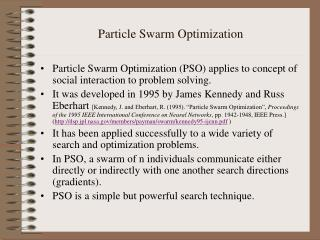DownloadDownload PresentationParticle Swarm Optimization

# Particle Swarm Optimization

Télécharger la présentation## Particle Swarm Optimization

- - - - - - - - - - - - - - - - - - - - - - - - - - - E N D - - - - - - - - - - - - - - - - - - - - - - - - - - -
##### Presentation Transcript

1. Particle Swarm Optimization • Particle Swarm Optimization (PSO) applies to concept of social interaction to problem solving. • It was developed in 1995 by James Kennedy and Russ Eberhart [Kennedy, J. and Eberhart, R. (1995). “Particle Swarm Optimization”, Proceedings of the 1995 IEEE International Conference on Neural Networks, pp. 1942-1948, IEEE Press.] (http://dsp.jpl.nasa.gov/members/payman/swarm/kennedy95-ijcnn.pdf ) • It has been applied successfully to a wide variety of search and optimization problems. • In PSO, a swarm of n individuals communicate either directly or indirectly with one another search directions (gradients). • PSO is a simple but powerful search technique.

2. Particle Swarm Optimization:Swarm Topology • In PSO, there have been two basic topologies used in the literature • Ring Topology (neighborhood of 3) • Star Topology (global neighborhood) I0 I0 I1 I1 I4 I4 I3 I2 I3 I2

3. Particle Swarm Optimization:The Anatomy of a Particle • A particle (individual) is composed of: • Three vectors: • The x-vector records the current position (location) of the particle in the search space, • The p-vector records the location of the best solution found so far by the particle, and • The v-vector contains a gradient (direction) for which particle will travel in if undisturbed. • Two fitness values: • The x-fitness records the fitness of the x-vector, and • The p-fitness records the fitness of the p-vector. Ik X = <xk0,xk1,…,xkn-1> P = <pk0,pk1,…,pkn-1> V = <vk0,vk1,…,vkn-1> x_fitness = ? p_fitness = ?

4. Particle Swarm Optimization:Swarm Search • In PSO, particles never die! • Particles can be seen as simple agents that fly through the search space and record (and possibly communicate) the best solution that they have discovered. • So the question now is, “How does a particle move from on location in the search space to another?” • This is done by simply adding the v-vector to the x-vector to get another x-vector (Xi = Xi + Vi). • Once the particle computes the new Xi it then evaluates its new location. If x-fitness is better than p-fitness, then Pi = Xi and p-fitness = x-fitness.

5. Particle Swarm Optimization:Swarm Search • Actually, we must adjust the v-vector before adding it to the x-vector as follows: • vid = vid + 1*rnd()*(pid-xid) + 2*rnd()*(pgd-xid); • xid = xid + vid; • Where i is the particle, • 1,2 are learning rates governing the cognition and social components • Where g represents the index of the particle with the best p-fitness, and • Where d is the dth dimension.

6. Particle Swarm Optimization:Swarm Search • Intially the values of the velocity vectors are randomly generated with the range [-Vmax, Vmax] where Vmax is the maximum value that can be assigned to any vid.

7. Particle Swarm Optimization:Swarm Types • In his paper, [Kennedy, J. (1997), “The Particle Swarm: Social Adaptation of Knowledge”, Proceedings of the 1997 International Conference on Evolutionary Computation, pp. 303-308, IEEE Press.] • Kennedy identifies 4 types of PSO based on 1 and 2 . • Given: vid = vid + 1*rnd()*(pid-xid) + 2*rnd()*(pgd-xid); xid = xid + vid; • Full Model (1, 2 > 0) • Cognition Only (1 > 0 and 2 = 0), • Social Only (1 = 0 and 2 > 0) • Selfless (1 = 0, 2 > 0, and g  i)

8. Particle Swarm Optimization:Related Issues • There are a number of related issues concerning PSO: • Controlling velocities (determining the best value for Vmax), • Swarm Size, • Neighborhood Size, • Updating X and Velocity Vectors, • Robust Settings for (1 and 2), • An Off-The-Shelf PSO • Carlisle, A. and Dozier, G. (2001). “An Off-The-Shelf PSO”, Proceedings of the 2001 Workshop on Particle Swarm Optimization, pp. 1-6, Indianapolis, IN. (http://antho.huntingdon.edu/publications/Off-The-Shelf_PSO.pdf)

9. Particle Swarm:Controlling Velocities • When using PSO, it is possible for the magnitude of the velocities to become very large. • Performance can suffer if Vmax is inappropriately set. • Two methods were developed for controlling the growth of velocities: • A dynamically adjusted inertia factor, and • A constriction coefficient.

10. Particle Swarm Optimization:The Inertia Factor • When the inertia factor is used, the equation for updating velocities is changed to: • vid = *vid + 1*rnd()*(pid-xid) + 2*rnd()*(pgd-xid); • Where  is initialized to 1.0 and is gradually reduced over time (measured by cycles through the algorithm).

11. Particle Swarm Optimization:The Constriction Coefficient • In 1999, Maurice Clerc developed a constriction Coefficient for PSO. • vid = K[vid + 1*rnd()*(pid-xid) + 2*rnd()*(pgd-xid)]; • Where K = 2/|2 -  - sqrt(2 - 4)|, •  = 1 + 2, and •  > 4.

12. Particle Swarm Optimization:Swarm and Neighborhood Size • Concerning the swarm size for PSO, as with other ECs there is a trade-off between solution quality and cost (in terms of function evaluations). • Global neighborhoods seem to be better in terms of computational costs. The performance is similar to the ring topology (or neighborhoods greater than 3). • There has been little research on the effects of swarm topology on the search behavior of PSO.

13. Particle Swarm Optimization:Particle Update Methods • There are two ways that particles can be updated: • Synchronously • Asynchronously • Asynchronous update allows for newly discovered solutions to be used more quickly • The asynchronous update method is similar to ____. I0 I1 I4 I3 I2